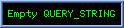Translated from the german web site :http://www.sprut.de/electronic/pic/projekte/elko/elko.htmThe simple capacitance measuring circuit was added to a low-impedance (25 ohms) push-pull power amplifier and a precision measuring rectifier to measure the ESR can.Particularly for use in switching power supplies are required with a small ESR capacitors. Since the ESR is frequency dependent, it is important to determine the ESR (100 kHz) at a sufficiently high frequency. are often in data sheets for small-to-findESR frequencies are useless for this purpose.The measurement of the ESR is complicated by the fact that the capacitor with its AC resistance / impedance Z) in series with the ESR is (. It is best to measure the total internal resistance (Rg) of the electrolytic capacitor at 100 kHz, and then deduct from that given by ELKO-capacity AC resistance. The total internal resistance (Rg) of the electrolytic capacitors in the range of 25 .. 500 Milliohm (bei 100kHz). 500 milliohms (at 100kHz). A measurement with a resolution of 5 milliohms is therefore appropriate. For measurement, a 100-kHz alternating current (5V pp, square wave) is sent to a series connection of a resistor (25 ohms) and the ELKO. The AC voltage drop across the electrolytic capacitor is measured and used to calculate the total internal resistance. A possible large current simplifies the measurement. At 100-mA AC current at the fall ELKO AC voltages of 2.5 .. 50 mVAC. In order to measure with a resolution of 0.25 mVAC, a precision rectifier is required, which rectifies the AC component of the voltage on the capacitor and at least 20-fold increase. Then the voltage can be measured with the ADC of a PIC. But in order to minimize the demands on the reference voltage of the ADCs, the measurement voltage better than 80-fold increase.With the 8 MSB of the ADC result can then total resistance of Rg = 2.5 .. 640 mOhm measure.The AC impedance Z is then calculated as follows: Z = 1 / (6.28 x F x C) = 1 / (628 318 x C) of reading and Rg taken off. ESR = Rg - ZThe 100-mA AC generated by the PIC (RC1, RC2), and with a push-pull driver stage (T1, T2) and 25 ohm resistors (R8, R9) increased.